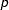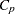The REG Procedure
 Model-Selection Methods

The nine methods of model selection implemented in PROC REG are specified with the SELECTION= option in the MODEL statement. Each method is discussed in this section.

### Full Model Fitted (NONE)

This method is the default and provides no model selection capability. The complete model specified in the MODEL statement is used to fit the model. For many regression analyses, this might be the only method you need.

### Forward Selection (FORWARD)

The forward-selection technique begins with no variables in the model. For each of the independent variables, the FORWARD method calculatesstatistics that reflect the variable’s contribution to the model if it is included. The-values for thesestatistics are compared to the SLENTRY= value that is specified in the MODEL statement (or to 0.50 if the SLENTRY= option is omitted). If nostatistic has a significance level greater than the SLENTRY= value, the FORWARD selection stops. Otherwise, the FORWARD method adds the variable that has the largeststatistic to the model. The FORWARD method then calculatesstatistics again for the variables still remaining outside the model, and the evaluation process is repeated. Thus, variables are added one by one to the model until no remaining variable produces a significantstatistic. Once a variable is in the model, it stays.

### Backward Elimination (BACKWARD)

The backward elimination technique begins by calculatingstatistics for a model which includes all of the independent variables. Then the variables are deleted from the model one by one until all the variables remaining in the model producestatistics significant at the SLSTAY= level specified in the MODEL statement (or at the 0.10 level if the SLSTAY= option is omitted). At each step, the variable showing the smallest contribution to the model is deleted.

### Stepwise (STEPWISE)

The stepwise method is a modification of the forward-selection technique and differs in that variables already in the model do not necessarily stay there. As in the forward-selection method, variables are added one by one to the model, and thestatistic for a variable to be added must be significant at the SLENTRY= level. After a variable is added, however, the stepwise method looks at all the variables already included in the model and deletes any variable that does not produce anstatistic significant at the SLSTAY= level. Only after this check is made and the necessary deletions are accomplished can another variable be added to the model. The stepwise process ends when none of the variables outside the model has anstatistic significant at the SLENTRY= level and every variable in the model is significant at the SLSTAY= level, or when the variable to be added to the model is the one just deleted from it.

### Maximum RImprovement (MAXR)

The maximumimprovement technique does not settle on a single model. Instead, it tries to find the "best" one-variable model, the "best" two-variable model, and so forth, although it is not guaranteed to find the model with the largestfor each size.

The MAXR method begins by finding the one-variable model producing the highest. Then another variable, the one that yields the greatest increase in, is added. Once the two-variable model is obtained, each of the variables in the model is compared to each variable not in the model. For each comparison, the MAXR method determines if removing one variable and replacing it with the other variable increases. After comparing all possible switches, the MAXR method makes the switch that produces the largest increase in. Comparisons begin again, and the process continues until the MAXR method finds that no switch could increase. Thus, the two-variable model achieved is considered the "best" two-variable model the technique can find. Another variable is then added to the model, and the comparing-and-switching process is repeated to find the "best" three-variable model, and so forth.

The difference between the STEPWISE method and the MAXR method is that all switches are evaluated before any switch is made in the MAXR method. In the STEPWISE method, the "worst" variable might be removed without considering what adding the "best" remaining variable might accomplish. The MAXR method might require much more computer time than the STEPWISE method.

### Minimum R(MINR) Improvement

The MINR method closely resembles the MAXR method, but the switch chosen is the one that produces the smallest increase in. For a given number of variables in the model, the MAXR and MINR methods usually produce the same "best" model, but the MINR method considers more models of each size.

### RSelection (RSQUARE)

The RSQUARE method finds subsets of independent variables that best predict a dependent variable by linear regression in the given sample. You can specify the largest and smallest number of independent variables to appear in a subset and the number of subsets of each size to be selected. The RSQUARE method can efficiently perform all possible subset regressions and display the models in decreasing order ofmagnitude within each subset size. Other statistics are available for comparing subsets of different sizes. These statistics, as well as estimated regression coefficients, can be displayed or output to a SAS data set.

The subset models selected by the RSQUARE method are optimal in terms offor the given sample, but they are not necessarily optimal for the population from which the sample is drawn or for any other sample for which you might want to make predictions. If a subset model is selected on the basis of a largevalue or any other criterion commonly used for model selection, then all regression statistics computed for that model under the assumption that the model is given a priori, including all statistics computed by PROC REG, are biased.

While the RSQUARE method is a useful tool for exploratory model building, no statistical method can be relied on to identify the "true" model. Effective model building requires substantive theory to suggest relevant predictors and plausible functional forms for the model.

The RSQUARE method differs from the other selection methods in that RSQUARE always identifies the model with the largestfor each number of variables considered. The other selection methods are not guaranteed to find the model with the largest. The RSQUARE method requires much more computer time than the other selection methods, so a different selection method such as the STEPWISE method is a good choice when there are many independent variables to consider.

### Adjusted RSelection (ADJRSQ)

This method is similar to the RSQUARE method, except that the adjustedstatistic is used as the criterion for selecting models, and the method finds the models with the highest adjustedwithin the range of sizes.

### Mallows’ CSelection (CP)

This method is similar to the ADJRSQ method, except that Mallows’statistic is used as the criterion for model selection. Models are listed in ascending order of.